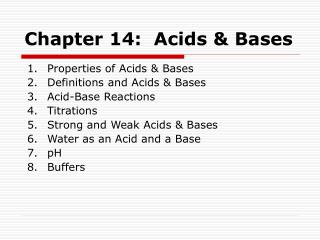DownloadDownload PresentationChapter 14: Acids & Bases

# Chapter 14: Acids & Bases

Download Presentation## Chapter 14: Acids & Bases

- - - - - - - - - - - - - - - - - - - - - - - - - - - E N D - - - - - - - - - - - - - - - - - - - - - - - - - - -
##### Presentation Transcript

1. Chapter 14: Acids & Bases • Properties of Acids & Bases • Definitions and Acids & Bases • Acid-Base Reactions • Titrations • Strong and Weak Acids & Bases • Water as an Acid and a Base • pH • Buffers

2. Example 1 Write the formula for the conjugate base of each of the following acids: • HNO3 c. H3PO4 • HCl d. H2O Write the formula for the conjugate acid of each of the following bases: • C2H3O3– c. PO43- • H2O d. NH3

3. Example 2 Write the products for each reaction below and balance the equation. • HF (aq) + Sr(OH)2(aq)  • HNO3(aq) + KHCO3(aq)  • HI(aq) + Ca(s)  • HCl(aq) + K2O(s) 

4. Example 3 A student titrates a 25.00 mL sample of a solution of H2SO4 of uknown concentration. If it takes 27.33 mL of 0.1129 M KOH to reach the equivalence point, what was the molarity of the original H2SO4 solution?

5. Example 4 Calculate [H+] and [OH–]for each of the following solutions: • A solution that is 2.0×10-5 M H+. • A solution that is 1.1×10-2 M OH–. • A 0.10 M solution of HBr.

6. Example 5 Calculate the pH and pOH of each of the following solutions: • A solution that is 2.2×10-3 M H+. • A solution that is 5.0×10-5 M OH–. • A 0.15 M solution of HNO3.

7. Example 6 Calculate [H+] and [OH–]for each of the following solutions: • A solution with a pH of 2.5 • A solution with a pH of 10.2 • A solution with a pOH of 3.3 • A solution with a pOH of 11.0

8. Example 7 Write the equation for the reaction that occurs when HNO3 is added to a buffer made of KC2H3O2 and HC2H3O2. Write the equation for the reaction that occurs when KOH is added to the same buffer.